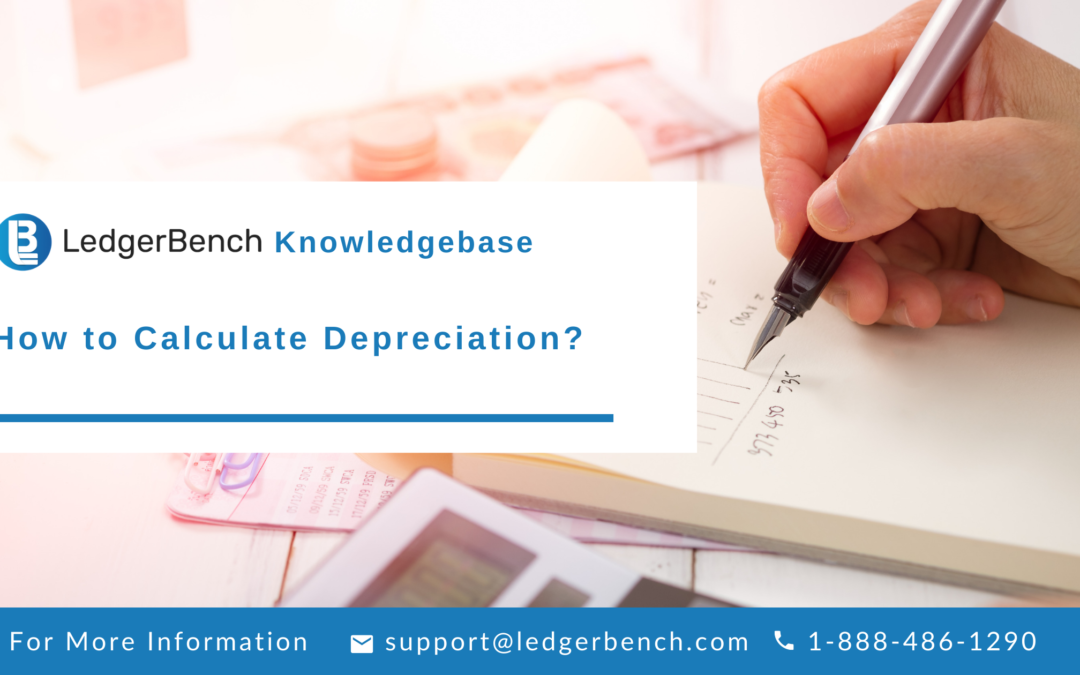1-866-852-0889 support@ledgerbench.com
Select Page## How to Calculate Depreciation?

To calculate depreciation, subtract the asset’s salvage value from that of the cost to determine the amount of depreciation. Divide this amount with the number of asset’s useful lifespan. Now, divide it by 12 to get the monthly depreciation for the asset.

Depreciation refers to the value of a business asset over its useful life.

## Following is the information you may need to calculate depreciation:

• Useful life of the asset:

This information is available in the form of a table based on the type of asset. For the value of a specific asset, you will require an accountant to tell you the useful life of an asset.

• Minus the salvage value:

You need to minus the salvage value of the asset at the end of its  useful life. Just like the useful life, salvage value is determined by the table.

You May Also Read – What Does a Bookkeeper Do for a Small Business?

• Divided by the cost of the asset:

This includes all costs to acquire the assets such as transportation cost, setting up and training.

All this will result in the book value of the asset.

For instance, the annual depreciation on a machinery with a useful life of 10 years, a salvage value of \$1000 and a cost of \$1,00000 is \$9900 (1,00000 – \$1000)/ 10

The asset must be placed in service in the first year that depreciation is calculated, for accounting and tax purposes.

For assets purchased in the middle of the year, the annual depreciation expense is divided by the number of months in that year since the purchase.

### What is Accumulated Depreciation:

The cumulative depreciation of an asset at a single point in its life is known as Accumulated Depreciation. The carrying value of an asset on a balance sheet is the difference between its purchase price and accumulated depreciation. A business buys and holds an asset on the balance sheet until the salvage value matches the carrying value.

Accumulated Depreciation is applicable to assets that are capitalized. Capitalized assets are those assets which provide value for more than one year.

Accounting rule states that expenses and sales are matched in the period in which they have incurred. Depreciation is the solution for this matching problem for capitalized assets.

A portion of the cost of the assets in the year it is purchased and for the rest of the assets useful life is considered a depreciation expense. Accumulated depreciation is the total amount that the asset has been depreciated over the asset’s life.

Suggested Reads – Common Balance sheet mistakes and how to avoid them

### Three main methods of calculating Depreciation:

Depreciation can be calculated in two different ways on a monthly basis:

Determining monthly accumulated depreciation for an asset depends on the asset’s useful lifespan as determined by the IRS and accounting method used by you.

The useful lifespan of an asset can range from 3 to 20 years for personal property, 39 years for business real estate, 15 to 20 years for land improvements and 27.5 years for residential real estate. The IRS has information for depreciation as well as life span of an asset.

The IRS is currently using the Modified Accelerated Cost Recovery System (MACRS)

It is the depreciation system that allows depreciation to be calculated either by the straight line method or the declining balance method.

#### Straight Line Method:

To use the straight line method, you choose to depreciate your property at an annual amount for each over its useful lifespan.

Annual depreciation = Depreciation factor * 1/lifespan * remaining book value

#### Use the following steps to calculate straight line method:

1. Subtract the assets salvage value from its cost to determine the amount that can be depreciated
2. Divide this amount by the number of years in the asset’s useful lifespan
3. Divide by 12 to calculate the monthly depreciation on assets.

Suggested Reads – IRS Tax Relaxation Deadlines for Covid-19 (With State Regulations)

#### Declining Balance Method:

This method is used to recognize the majority of an asset’s depreciation early in it’s lifespan. There are two variations in this:

The double declining method and the 150% declining balance method.

The depreciation changes every year and hence it is more difficult to calculate than the straight line method.

The following formula is used to calculate depreciation using declining method:

To convert this to yearly depreciation divide this result by 12.

Annual depreciation = Depreciation factor * 1/Lifespan * remaining book value

#### SUM-OF-THE-YEARS’-DIGITS (SYD) METHOD:

The method that takes an asset’s expected life and adds together the digits for each year is known as the sum of the years digit (SYD) method. This is an accelerated method to calculate depreciation.

So, if the asset is expected to last for five years, then the sum of the year’s digit would be calculated by adding 5+4+3+2+1 to get the sum of 15. Each digit is then divided by this sum to determine the percentage by which assets should be depreciated each year, starting with the highest number each year which is 1.

#### The formula to calculate depreciation by using SYD method is:

Depreciation expense = Remaining useful life of the asset/ sum of the years digit * depreciable cost